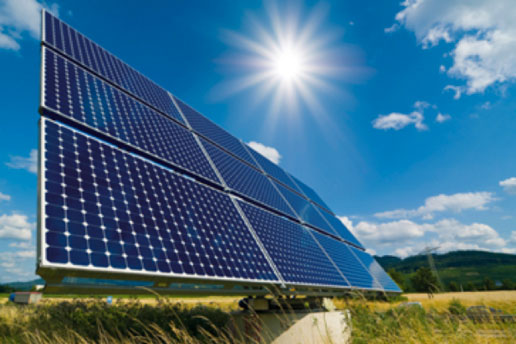# Hydro PowerINTRODUCTION:

1. Hydroelectric power (hydropower) systems convert the kinetic energy in flowing water into electric energy.

2. Falling or flowing water turns a propeller like piece called a turbine.

3.The turbine turns a metal shaft in an electric generator which produces electricity.

1. How a Hydroelectric Power System Works?
2. Flowing water is directed at a turbine.
3. The flowing water causes the turbine to rotate, converting the water’s kinetic energy into mechanical energy.
4.  The mechanical energy produced by the turbine is converted into electric energy using a turbine generator. Inside the generator, the shaft of the turbine spins a magnet inside coils of copper wire.
5. It is a fact of nature that moving a magnet near a conductor causes an electric current.
1.